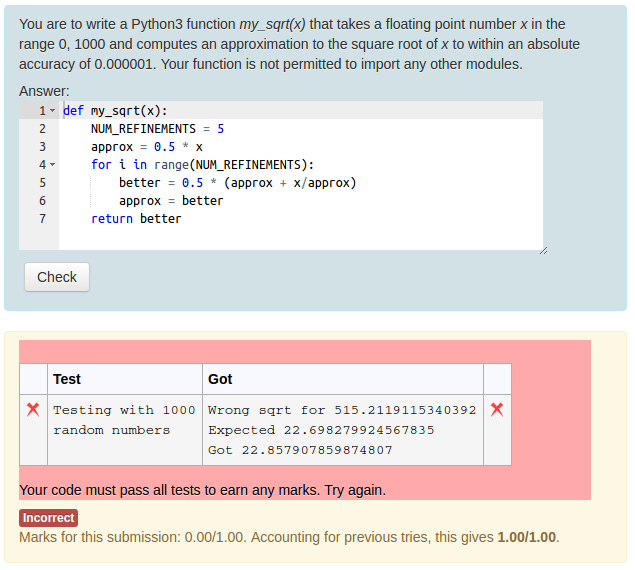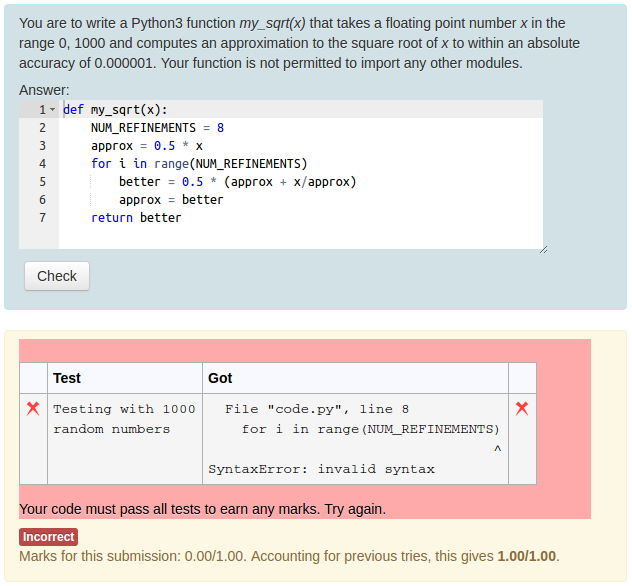## CodeRunner Documentation (V3.0.0)

### 9.2 A more advanced grading-template example

A template-grader can also be used to grade programming questions when the usual graders (e.g. exact or regular-expression matching of the program's output) are inadequate.

As a simple example, suppose the student has to write their own Python square root function (perhaps as an exercise in Newton Raphson iteration?), such that their answer, when squared, is within an absolute tolerance of 0.000001 of the correct answer. To prevent them from using the math module, any use of an import statement would need to be disallowed but we'll ignore that aspect in order to focus on the grading aspect.

The simplest way to deal with this issue is to write a series of testcases of the form

    approx = student_sqrt(2)
if math.abs(approx - right_answer) < 0.00001:
print("OK")
else:
print("Fail (got {}, expected {})".format(approx, right_answer))


where the expected output is "OK". However, if one wishes to test the student's code with a large number of values - say 100 or more - this approach becomes impracticable. For that, we need to right our own tester, which we can do using a template grade.

Template graders that run student-supplied code are somewhat tricky to write correctly, as they need to output a valid JSON record under all situations, handling problems like extraneous output from the student's code, runtime errors or syntax error. The safest approach is usually to run the student's code in a subprocess and then grade the output.

A per-test template grader for the student square root question, which tests the student's student_sqrt function with 1000 random numbers in the range 0 to 1000, might be as follows:

    import subprocess, json, sys
student_func = """{{ STUDENT_ANSWER | e('py') }}"""

if 'import' in student_func:
output = 'The word "import" was found in your code!'
result = {'got': output, 'fraction': 0}
print(json.dumps(result))
sys.exit(0)

test_program = """import math
from random import uniform
TOLERANCE = 0.000001
NUM_TESTS = 1000
{{ STUDENT_ANSWER | e('py') }}
ok = True
for i in range(NUM_TESTS):
x = uniform(0, 1000)
right = math.sqrt(x)
if abs(right - stud_answer) > TOLERANCE:
print("Wrong sqrt for {}. Expected {}, got {}".format(x, right, stud_answer))
ok = False
break

if ok:
print("All good!")
"""
try:
with open('code.py', 'w') as fout:
fout.write(test_program)
output = subprocess.check_output(['python3', 'code.py'],
stderr=subprocess.STDOUT, universal_newlines=True)
except subprocess.CalledProcessError as e:
output = e.output

mark = 1 if output.strip() == 'All good!' else 0
result = {'got': output, 'fraction': mark}
print(json.dumps(result))


The following figures show this question in action.Obviously, writing questions using custom graders is much harder than using the normal built-in equality based grader. It is usually possible to ask the question in a different way that avoids the need for a custom grader. In the above example, you would have to ask yourself if it mightn't have been sufficient to test the function with 10 fixed numbers in the range 0 to 1000 using ten different test cases of the type suggested in the third paragraph of this section.